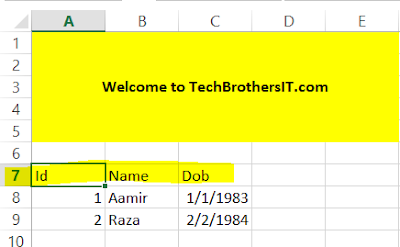### C# - How to Read Excel Sheet Data after Skipping Rows and Load to SQL Server Table in C#

You are working as C# developer or Dot Net Developer. You need to write a program that should be able to skip some rows from an Excel Sheet and load the remaining records to SQL Server Table. The program should be able to handle to load multiple Excel files.

Here is my sample excel sheet with some data.How to Skip Rows in Excel Sheet and Export actual records to SQL Server Table in C#

As you can see that I have first 5 rows as company name. In your case you might have logo or any information. In my case, I need to start reading from A7 and load the data to dbo.Customer table.

We also validate the columns against our Table columns in database, let's say if there is a column in excel and it does not exists in database table, the program is going to ignore that column and insert the data for the columns which are present in excel and database table.

Let's create customer table first and then write C# code to skip the rows and load the data to table.

```CREATE TABLE [dbo].[Customer](
[Id] [int] NULL,
[Name] [varchar](100) NULL,
[Dob] [date] NULL
)```

I created Console Application by using below C# code.

```using System;
using System.Collections.Generic;
using System.Linq;
using System.Text;
using System.IO;
using System.Data;
using System.Data.OleDb;
using System.Data.SqlClient;

namespace TechBrothersIT.com_CSharp_Tutorial
{
class Program
{
static void Main(string[] args)
{
//the datetime and Log folder will be used for error log file in case error occured
string datetime = DateTime.Now.ToString("yyyyMMddHHmmss");
string LogFolder = @"C:\Log\";
try
{
//Provide the path where Excel files exists
string FolderPath = @"C:\Source\";
//Provide the table name in which you want to load the data
string TableName = "Customer";
//Provide the schema of table
string SchemaName = "dbo";
//Provide the starting column for read actul records
string StartingColumn = "A";
//Provide the end column till which you want to read
string EndingColumn = "C";
//Provide the row number from which you like to start reading
//Provide the Database Name in which table or view exists
string DatabaseName = "TechbrothersIT";
//Provide the SQL Server Name
string SQLServerName = "(local)";
var directory = new DirectoryInfo(FolderPath);
FileInfo[] files = directory.GetFiles();

//Declare and initilize variables
string fileFullPath = "";

//Get one Book(Excel file at a time)
foreach (FileInfo file in files)
{
fileFullPath = FolderPath + "\\" + file.Name;

//Create Excel Connection
string ConStr;
string HDR;
HDR = "YES";
ConStr = "Provider=Microsoft.ACE.OLEDB.12.0;Data Source="
+ fileFullPath + ";Extended Properties=\"Excel 12.0;HDR=" + HDR + ";IMEX=0\"";
OleDbConnection cnn = new OleDbConnection(ConStr);

//Get Sheet Name, it can handle multiple sheets
cnn.Open();
DataTable dtSheet = cnn.GetOleDbSchemaTable(OleDbSchemaGuid.Tables, null);
string sheetname;
sheetname = "";
foreach (DataRow drSheet in dtSheet.Rows)
{
if (drSheet["TABLE_NAME"].ToString().Contains("\$"))
{
sheetname = drSheet["TABLE_NAME"].ToString();

//Load the DataTable with Sheet Data so we can get the column header
OleDbCommand oconn = new OleDbCommand("select top 1 * from ["
+ sheetname + StartingColumn + StartReadingFromRow + ":" + EndingColumn + "]", cnn);
DataTable dt = new DataTable();
cnn.Close();

//Prepare Header columns list so we can run against Database to get matching columns for a table.
//If columns does not exists in table, it will ignore and load only matching columns data
string SQLQueryToGetMatchingColumn = "";
for (int i = 0; i < dt.Columns.Count; i++)
{
if (i != dt.Columns.Count - 1)
ExcelHeaderColumn += "'" + dt.Columns[i].ColumnName + "'" + ",";
else
ExcelHeaderColumn += "'" + dt.Columns[i].ColumnName + "'";
}

SQLQueryToGetMatchingColumn = "select STUFF((Select  ',['+Column_Name+']' from Information_schema.Columns where Table_Name='" +
TableName + "' and Table_SChema='" + SchemaName + "'" +
"and Column_Name in (" + @ExcelHeaderColumn + ") for xml path('')),1,1,'') AS ColumnList";

//Create Connection to SQL Server Database from which you like to export tables to Excel
SqlConnection SQLConnection = new SqlConnection();
SQLConnection.ConnectionString = "Data Source = "
+ SQLServerName + "; Initial Catalog ="
+ DatabaseName + "; "
+ "Integrated Security=true;";

//Get Matching Column List from SQL Server
string SQLColumnList = "";
SqlCommand cmd = SQLConnection.CreateCommand();
cmd.CommandText = SQLQueryToGetMatchingColumn;
SQLConnection.Open();
SQLColumnList = (string)cmd.ExecuteScalar();
SQLConnection.Close();

//Use Actual Matching Columns to get data from Excel Sheet
OleDbConnection cnn1 = new OleDbConnection(ConStr);
cnn1.Open();
OleDbCommand oconn1 = new OleDbCommand("select " + SQLColumnList
+ " from [" + sheetname + StartingColumn
+ StartReadingFromRow + ":" + EndingColumn + "]", cnn1);
DataTable dt1 = new DataTable();
cnn1.Close();

SQLConnection.Open();
//Load Data from DataTable to SQL Server Table.
using (SqlBulkCopy BC = new SqlBulkCopy(SQLConnection))
{
BC.DestinationTableName = SchemaName + "." + TableName;
foreach (var column in dt1.Columns)
BC.WriteToServer(dt1);
}
SQLConnection.Close();

}
}
}
}

catch (Exception exception)
{
// Create Log File for Errors
using (StreamWriter sw = File.CreateText(LogFolder
+ "\\" + "ErrorLog_" + datetime + ".log"))
{
sw.WriteLine(exception.ToString());

}

}

}
}
}```

I executed above program and it was able to skip the rows in excel sheet and loaded the actual data according as by variable values I have provided at start of program.How to skip rows in Excel and import actual data rows to SQL Server table in C#